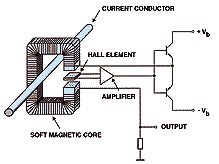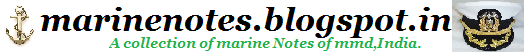Home » » How does a clamp meter work, How does a dc clampmeter work?

# How does a clamp meter work, How does a dc clampmeter work?

### How does a dc clampmeter work?Ordinary clampmeters used to measure AC currents work on the principle of electromagnetic induction caused by the alternating current flowing in the conductor which reverses direction causing a dynamically changing magnetic field. However, in DC conductors, the current flows in a fixedpolarity. Consequently, the magnetic field around the conductor is fixed and does not change. Hence, a conventional clamp meter will register no reading.

ADC clampmeter works on the principle of the Hall Effect. The Hall Effect, named after Edwin Hall who discovered it 1879, states that when a conductor carrying current is placed in a magnetic field, a potential is induced across the conductor, transverse to an electric current in the conductor and a magnetic field perpendicular to the current. It is caused as the chargecarriers, electrons or holes, experiencea force known as the Lorentz force and are pushed to the sides of the conductor.

A clampmeter which works onthe Hall effect has a sensor known as the Hall element.The Hall element is subjected to the magnetic field caused by the flow of current to be measured. This causes a small voltage across the Hall element. This voltage is amplified and measured.
How does a clamp meter work?
It is often very difficult to measure the current directly, since the value of the current is too large to apply it directly to the meter circuit, or simply because it is unacceptable to break the circuit.Clamp current sensors are designed to enhance the measuring possibilities of digital multimeters, power meters, oscilloscopes, portable oscilloscopes, recorders or self-recorders, and other various devices.A current sensor for measuring the parameters of alternating current can be regarded as a kind of a simple current transformer.On a closed magnetic circuit in the form of clamp that is closed to the conductor, there is the secondary coil, through which passes an electric current, induced by a current in the conductor under measurement.The conductor, where user holds the measurements, is one turn of primary winding.The current sensor, closed around the conductor, generates an output current, the value of which is directly proportional to the current in the conductor. Given a ratio of transformation, the value of current in the wire, around which the sensor is closed, can be easily determined.If the measurable value is too small, several loops of the conductor can be closed by the clamp, in order to enable the operation of the sensor, or to increase the measurement accuracy. The current value is determined by the ratio of the sensor indications to the number of turns of the conductor, covered by the clamp (sensor indications must be divided by the number of turns, closed by the clamp).Unlike traditional electromagnetic alternating current converters, the measurement of the parameters of alternating and direct currents is often performed by measuring the intensity of the magnetic field, created by the conductor in a semiconductor crystal in accordance with Hall effect.When the excitation current in the Hall-effect device is maintained to be constant, the intensity of the magnetic field is directly proportional to the current in the conductor under measurement. Thus, the output voltage corresponds to a given current.Such a circuit has two important advantages for the measurement of current:The first is that since the Hall voltage is independent of changes in the magnetic field direction. It depends only on the value of its intensity, thus, this device can be used to measure the direct current.The second advantage is that when the voltage of the magnetic field changes due to the changes in the current in the conductor, the ...